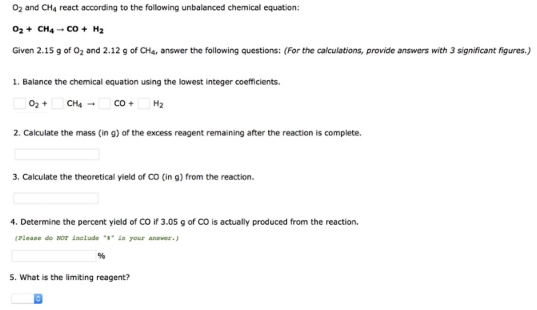# Problem: O2 and CH4 react according to the following unbalanced chemical equation: O2 + CH4 → __CO + __H2 Given 2.15 g of O2 and 2.12 g of CH4, answer the following questions: (For the calculations, provide answers with 3 significant figures.) 1. Balance the chemical equation using the lowest integer coefficients. __O2 + __CH4 → CO + H2. 2. Calculate the mass (in g) of the excess reagent remaining after the reaction is complete. 3. Calculate the theoretical yield of CO (in g) from the reaction. 4. Determine the percent yield of CO if 3.05 g of CO is actually produced from the reaction. (Please do NOT include in your answer.)5. What is the limiting reagent?

###### FREE Expert Solution
99% (349 ratings)###### Problem Details

O2 and CH4 react according to the following unbalanced chemical equation:

O2 + CH4 → __CO + __H2

Given 2.15 g of O2 and 2.12 g of CH4, answer the following questions: (For the calculations, provide answers with 3 significant figures.)

1. Balance the chemical equation using the lowest integer coefficients.

__O2 + __CH4 → CO + H2

2. Calculate the mass (in g) of the excess reagent remaining after the reaction is complete.

3. Calculate the theoretical yield of CO (in g) from the reaction.

4. Determine the percent yield of CO if 3.05 g of CO is actually produced from the reaction.